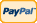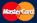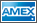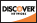# Pi Day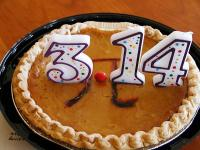For the first time “Pi” day was celebrated in 1988 in the Exploratorium museum of San Francisco.

On the West this event is celebrated at 1:59 pm to make an appropriate 3.14159 when combined with the date (in 12-hour system). Those who use 24-hour system consider that this time is 13:59 and therefore prefer to celebrate it at night.

Secret of Pi

Mathematicians have made a significant step trying to answer how much this figure is random. And for the first time they have managed to connect the number theory with the theory of chaos.

Pi value is known up to 500 billion of signs, its first figures are 3.1415926535. There is no any cyclic sequence in it and, if the mathematicians are not mistaken, it will never have one ever after.

Pi – circumference to diameter ratio  used to be considered mystical and ancient Greeks even had a special religion based on it. Any figures sequence of equal length can be seen in it with the same frequency. For example the possibility to find 234 sequence equals the possibility to find 876 and 23568 can be noticed as frequently as 98427. Mathematicians call such numbers normal. Other examples of normal numbers are: square root of 2 and natural logarithm of 2.

The feature of algorithm is that it works not with the full number but with its fragment. Scientists took the following numbers: 0.314; 0.141; 0.415; 0.159 etc. All of them are made of three consecutive figures of Pi number. If all of the Pi figures are random that means that all of those figures should be randomly placed between 0 and 1. Although the scientists worked not with decimal but binary notation of Pi, thus they worked with the sequence of 0 and 1.

The calculations made by the program of Bailey and his colleagues showed that Pi figures behave in accordance with the theory of chaos, that means that obviously their sequence is random. Possible applications of these results  new algorithm of random-number generator and cryptography.

Why is it March 14, 1:59?

In numerical term it begins with 3,141592 and has a constant mathematical duration.

Another variant is that date March 14 looks like 3.14, and it makes it clear why the celebration falls exactly on that very day.

As specialists consider this number was found by Babylonian magicians. It was used for the building of the famous Tower of Babel. However not exact value calculation of Pi number broke this project. Perhaps this mathematical constant was in the building basis of the legendary King Solomon’s Temple.

Another interesting fact is that Pi birthday coincides with the birthday of one of the most famous physicist  Albert Einstein.Back to the list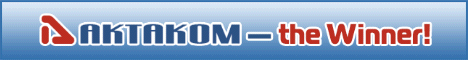Site map|Privacy policy|Terms of Use & Store Policies|How to Buy|Shipping|Payment|© T&M Atlantic, Inc., 2010-2023